# Functions Basic - Excel 2013

## Introduction

A formula is an expression which calculates the value of one or more cell(s). The functions are also predefined formulas and it can be used independently in Excel and performs calculations using specific values in a particular order. A simple formula can be created using add, subtract, multiply or divide values in your worksheet. Formulas always start with an equal sign (=), followed by constants that are numeric values and calculation operators such as plus (+), minus (-), asterisk(*), or forward slash (/) signs.

Example:

Cell B4 below contains a formula which adds the value of cell B1, B2 and B3.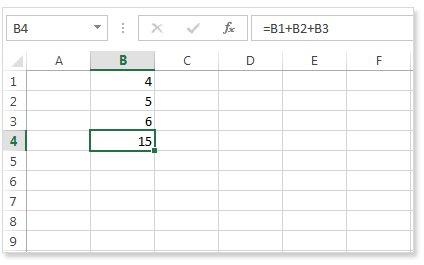The example below shows that the cell B4 contains the SUM function which calculates the sum of the range B1 to B3.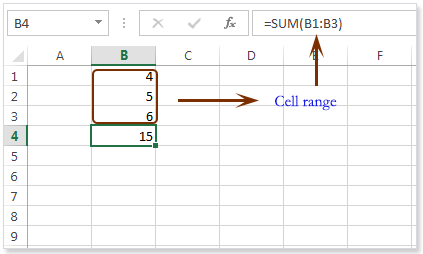How Enter a Formula ?

To enter a formula, execute the following steps.

Select a cell.

If you want to enter a formula, type an equal sign (=).

Example : Type the formula =B4 B1+B2+B3 into the cell B4.Instead of typing B1, B2, and B3, you can type an '=' sign first therefore simply select click or select the cell you want to add and type '+' operator between two selection.

Change the value of cell B1 to 8 and see the change of the result.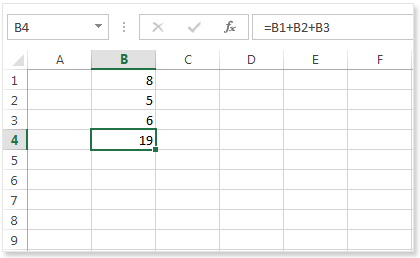How Edit a Formula ?

When you select a cell which contains a formula, Excel shows the value or formula of the cell in the formula bar.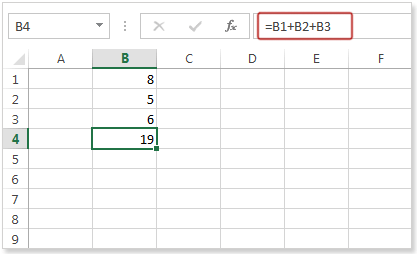To edit a formula, click in the formula bar and change the formula.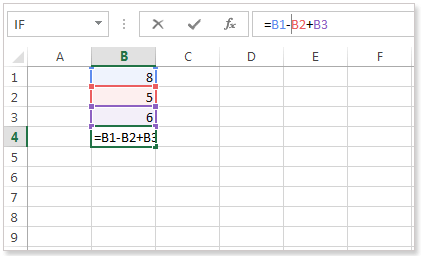Press Enter, and the change the of the output.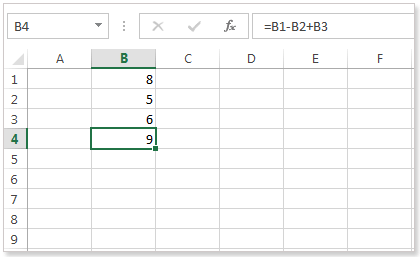## Operator Precedence

Excel uses a default order in which calculations occur. If a part of the formula is in parentheses, that part will be calculated first. It then performs multiplication or division calculations. Once this is complete, Excel will add and subtract the remainder of the formula. Here is the example below.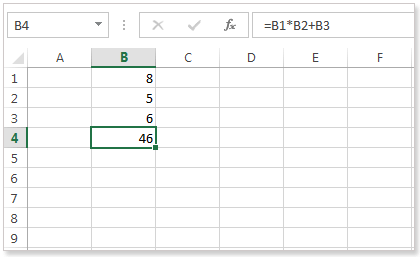In the above formula excel first, performs multiplication (B1 * B2). Next, Excel adds the value of cell B3 to this result.

Here is another example below.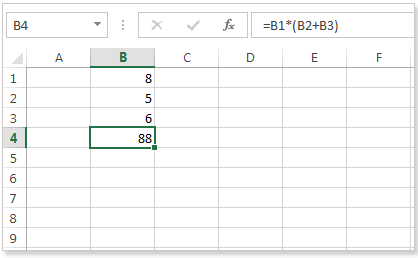In the above example, excel first calculates the part in parentheses (B2+B3). Next, it multiplies this result by the value of cell B1.

## Copy/Paste a Formula

When you copy a formula, Excel automatically changes the cell references for each new cell the formula is copied to. Here are steps below.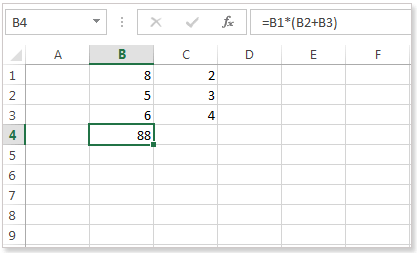Select cell B4, right-click, and then click Copy (or press CTRL + c) as shown below.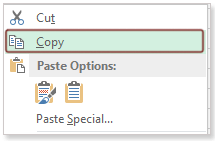Next, select cell the C4 and right-click, and then click Paste under 'Paste Options:' (or press CTRL + v).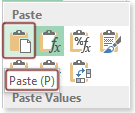You can also drag the formula horizontally or vertically according to your data in the sheet. Here in the picture below shows that select cell B4, click on the lower right corner of cell B4 and drag it across to cell C4.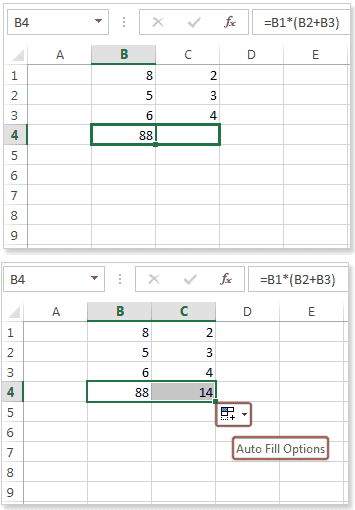Result. The formula in cell C4 references the values in column C.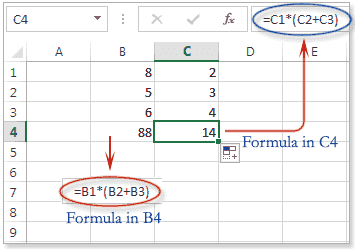## Insert a Function

Every function has a structure. Excel includes many common functions that can be useful for quickly finding the sum, average,count, maximum value, and minimum value for a range of cells.

A function must have a syntax which indicates how to write a specific function. The basic syntax for a function is an equals sign (=), then the function name (SUM, for example), and one or more arguments within parentheses. Arguments contain the information you want to calculate. Suppose, SUM(B1:B4). This function adds the values in cells B1,B2, B3, and B4. Here is the picture below shows the parts of the function.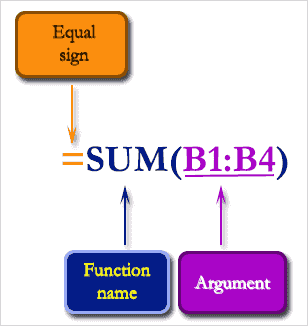Arguments :

Arguments may be a single cell or a range of cells and must be enclosed in parentheses. You can include one argument or multiple arguments, depending on the syntax required for the function.

For example, the function =SUM(B1:B3) would calculate the sum of the values in the cell range B1:B3. This function contains only one argument. Here is the picture below.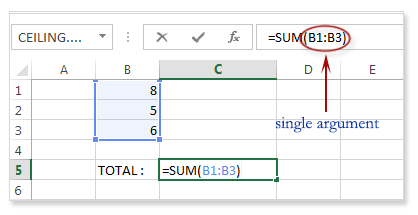Multiple arguments can be used but it must be separated by a comma. For example, the function =SUM(B1:B3, D1:D2,F1) will add the values of all the cells in the three arguments. Here is the picture below.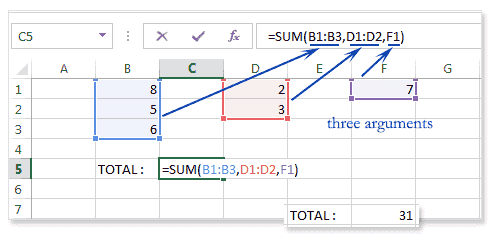Here are the steps below describes how to insert a function.

Select a cell D2 then click the Insert Function button.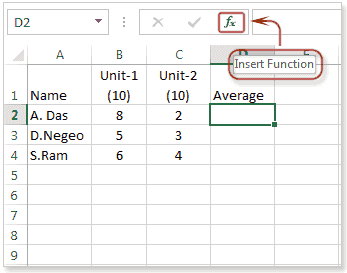The 'Insert Function' dialog box appears.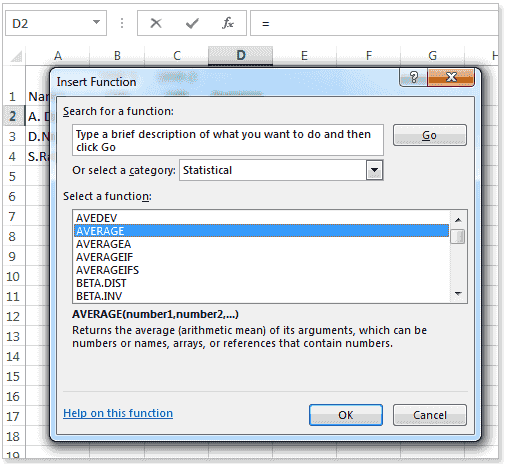Search for a function or select a function from a category. For example, choose AVERAGE from the Statistical category.

Then click ok

The 'Function Arguments' dialog box appears.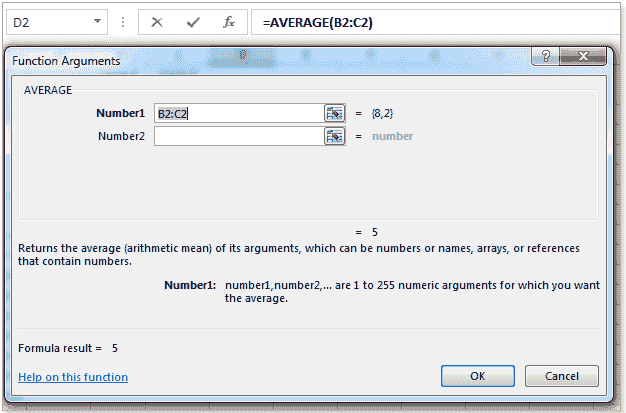Click Ok.

Output : Average of B2 and C2 is 5.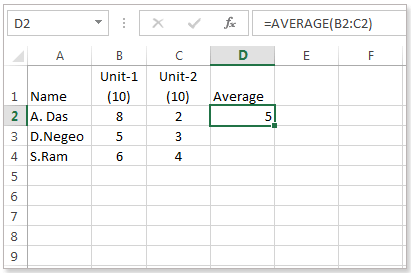Another way to write the function is to click D2 and simply type '=AVERAGE(B2:C2)' or Click B2 and drag upto C2, then press Enter key. Here is the picture below.﻿